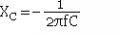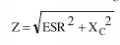# Calculating ripple current in a capacitor

#### Willnv

Joined Nov 24, 2018
12
I'm trying to figure out how to calculate ripple amperage but I cant find any good formulas for what I want to do. I want to smooth the output through a full bridge rectifier where the input is 240v ac and the current draw around 50 amps. The reason is because it was originally meant for 3 phase so it would have had alot less ripple then my single phase input. I found a formula for figuring out the ripple voltage.

So if I used a 18,000 uf capacitor such as this one https://www.mouser.ca/ProductDetail...Z1n0r9vR22YqjN7vGN3ZSV9u2P47iI0pNPC1xmjIBdg==

Using the formula I found:
Vripple = I / ffc
Vripple = 50 / (120 x 0.018)

I get 23v. Probably good enough for my application. But I cant figure out how to calculate the ripple current in the capacitor. One source seemed to indicate you could use roughly half the load current. So if load current is 50amps what would the ripple current be? That particular cap has a ripple current rating of 27.8A. If I put 2 of them in parallel does that double my ripple current? Is it possible for the ripple current be higher then what the load is?

#### DickCappels

Joined Aug 21, 2008
9,626
RMS current is RMS voltage divided by Z. Remember to include the effects of ESR on Z.

•ArakelTheDragon

#### Hymie

Joined Mar 30, 2018
1,115
The capacitor in your link has a maximum ripple current of 27.8A; therefore it will have a very short life with rectified 240V applied with a ripple current of 50A.

While the nominal DC voltage will be 340V, it is common to see 420V or 450V rated capacitors in such applications.

Given the above, I would recommend you configure two of these capacitors; 10,000uF/400V (https://www.mouser.co.uk/datasheet/2/293/e-lnu-1220691.pdf) in parallel to share the ripple current. With a temperature rating to 105C will also assist in maximising the capacitor’s life expectancy.

•Willnv

#### Willnv

Joined Nov 24, 2018
12
Given the above, I would recommend you configure two of these capacitors; 10,000uF/400V (https://www.mouser.co.uk/datasheet/2/293/e-lnu-1220691.pdf) in parallel to share the ripple current. With a temperature rating to 105C will also assist in maximising the capacitor’s life expectancy.
Thanks. I was also looking at these caps which have an even high rating but are quite a bit more money:
https://www.mouser.ca/ProductDetail...=sGAEpiMZZMtZ1n0r9vR22acXU6f7tU8qaFL/kKiDjdU=

RMS current is RMS voltage divided by Z
Thanks but how do I calculate Z? I found this calculator but when I plug in the numbers it doesn't seem to be right. https://www.allaboutcircuits.com/tools/capacitor-impedance-calculator/

For Instance if I put in a 18,000 uf at 120hz I get a value of 0.0737. Then 340/0.037 = 4, 613. That cant be right lol.

I also found another formula but it was in an article talking about tantalum capacitors so I dont know if its right either. The formula they gave is z = 1/2pi * f * C
Plug on the numbers for 120hz and 18, 000uf and I get z = 3.39
So then 340v/3.39 gives a value of 100 amps. Given that value I would probably need 3 of those caps. Sounds a little more reasonable but Im still confused. Can the ripple current be higher then what the load is?

#### ericgibbs

Joined Jan 29, 2010
17,174

#### Willnv

Joined Nov 24, 2018
12
That is pretty cool, I will have to try out that program. I'm not sure how I interpret the data though. Is that saying the current in the cap is 100amps?

#### DickCappels

Joined Aug 21, 2008
9,626
Z is the total impedance. That calculator calculates the capacitive reactiance, XZ = Square Root of (R squared + X squared).Since ESR is probably very small compared to Xc the the results of the two formulas shown here should agree pretty closely with the results of you calculator.

#### ericgibbs

Joined Jan 29, 2010
17,174
Thats what the sim is indicating.
Note the input current peaks.!!
E

#### ebp

Joined Feb 8, 2018
2,332
Impedance of the capacitor versus frequency is only useful if you know the actual frequency components of the current "pulses." The mains frequency is not instructive.

Simulation is definitely the best way to do it with any reasonable accuracy. It is NOT a simple problem because it is influenced by the source impedance and the equivalent series resistance (ESR) of the capacitor. Increasing either decreases the peak amplitude of the ripple current. ESR is now commonly specified for most capacitors that would be used in power applications. The source impedance is harder to estimate. If source impedance and ESR are both very low, then even the miscellaneous resistances such as the rectifiers and wiring may make a difference. The ripple of course does not all go "into" the capacitor but also directly into the load.

It is the RMS value of the ripple that is important because it is what acts on the ESR of the capacitor to produce heat and raise the temperature of the cap. Capacitor life approximately halves for each 10 °C rise in ambient temperature. Temperature rise due to ESR has an even stronger detrimental influence. The lower the circuit impedance, the narrower the charging current spikes and hence the higher the RMS to average ratio.

#### Willnv

Joined Nov 24, 2018
12
Thanks for all your help guys!
Im still a little confused by it all and the formulas Dick posted are still giving me huge values in the thousands of amps so Im not sure what I'm doing wrong.

Using the formula z = 1/2 * pi * f * C however is giving me a result very similar to Eric's simulation. Therefore putting a capacitor value in of 54, 000 μf ends up with a value of 17.93 amps. So am I safe to assume if I used 3 of the caps (ripple rating of 27.8 amps) I showed in the first post I would be good?

#### ebp

Joined Feb 8, 2018
2,332
Don't try to calculate using simple formulas with line frequency. You will not get meaningful numbers. Perhaps Eric will run the sim again to get the RMS value of the green curve. It will be an approximation that will be somewhat on the high side since he has no source impedance specified.

Making the capacitance higher actually increases the RMS current from the source.

ESR rises as capacitors age, so it is best to be quite conservative with initial selection.

#### ericgibbs

Joined Jan 29, 2010
17,174
hi ebp,
As you say you cannot use the line frequency.
Post #8 plot has the Icap RMS.
If the TS knows the source impedance of the supply I can include that.
E

#### ebp

Joined Feb 8, 2018
2,332
Hi, Eric
If the source is AC mains directly, its impedance will be very low but I have no quantitative feel for it. @MaxHeadRoom might have some useful input on that since he's done a lot of work with motor drives and the like.

It would be interesting to try it with a value equal the cap's ESR, just to see how doubling the resistance during charge would influence the magnitudes.

#### ericgibbs

Joined Jan 29, 2010
17,174
hi,
ESR 13mOhm gives 302Apk, 107A rms thru C1
ESR 26mOhm 273A pk, 103A rms

#### Willnv

Joined Nov 24, 2018
12
Eric would you mind do run the sim again with a capacitor value of 54, 000 uf( 3 of the 18, 000 uf caps in parallel)?

I really appreciate everyone's help on this but its all way over my head haha. Im just a welder with some basic understanding of electronics. Ive built some stuff out of arduino's but my understanding of all this electronic stuff is pretty limited.

As far as source impedance how do I know what that is? Does it have to do with the size (gauge) of wire feeding the rectifier? I can and will use a large supply, probably #4 copper.

#### ericgibbs

Joined Jan 29, 2010
17,174
hi W,
Using 3 off 18000uF , ESR 13m in parallel, this is what LTS outputs.
E

#### Willnv

Joined Nov 24, 2018
12
Thanks.

So getting better but still too high for those caps. Perhaps I will try that simulator and see if I can come up with some combination that will work for me.

Also as I understand the reason for the current rating is because the ripple heats up the cap shortening its life. How reasonable would it be to put those 3 caps on a large heat sink and running them at that current level?

#### Willnv

Joined Nov 24, 2018
12
I just noticed the 3 caps give a current value of about 1/3rd of the single one. I guess thats kindof what you would expect, they are sharing the amperage being that they are in parallel. So Id say its pretty safe to assume 4 of those would get me down to the "safe" level but still probably at their max rating. That's getting a bit expensive but still probably worth the expense for what I want to do.

Thank you all for the help

#### ericgibbs

Joined Jan 29, 2010
17,174
hi Will,
What is the actual application for this circuit.?
I see that you refer to a 3 phase conversion to 1 phase.
E